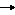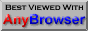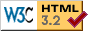## UNIT 4: Numeric Functions

### Introduction:

Functions represent a watershed in the study of BBC BASIC. On the one hand, they provide the opportunity to develop a wide range of new skills, particularly in speeding up operations on both numbers and strings. On the other hand, their syntax can be challenging for the relative beginner, and it is not uncommon for even the most enthusiastic student to find the going tough. A certain amount of patience will therefore needed to accept that with functions one is embarking on a chapter which almost deserves a book to itself.

To illustrate this, imagine that alongside your programming studies you were to work your way through a self-study book on elementary mathematics. You would soon find that functions which operate on numbers are so versatile, once you get to know them, that they could be applied to just about every mathematical problem in the book! With numeric functions, therefore, we get to the heart of the computer, in the literal sense of the word, as a tool for speeding up annotated calculation. Over and above that, however, BBC BASIC has the added feature of string functions, which can reduce complex operations on strings to relatively simple formulae.

Functions which operate on numbers are in fact automated "number-crunchers". Their key feature is that they produce a definite numeric result. It would not be wide of the mark to think of this "number-crunching machine" on the lines of a washing-machine: you put your numerical problems in, turn the dial to a given setting and get a definite result as the output. Admittedly this is a poetic image of what is going on. But it is more than that. The dial on a washing machine effectively selects different pre- programmed functions, in relation to factors such as time and temperature and speed of rotation, and the output from the selected functions gets converted into electrical signals, which then operate the mechanical devices in the machine. In the case of the personal computer, the output device which corresponds to the rotating drum in the washing machine is the screen or the printer.

Syntactically, functions are similar to procedures: they are usually "called" in the main body of the program, and are then "defined" after the END statement. (This is not always strictly necessary, but with functions, as with procedures, it is usually advisable to define the function after the END statement, so as to keep it self- contained in a complex program.) There is a potential drawback for the beginner in this arrangement, however, in that the syntax for calling the function, which occurs early in any program, does not make complete sense until you have linked it up to the definition of the function, which comes later, so the program has to be read several times over to make sense.

In the same way that the computer cannot use a variable until it has been defined, the programmer himself or herself cannot use a new routine in programming until it has itself been defined. So the plan in the following specimen programs, which illustrate simple addition, subtraction, multiplication and division, will be to study each program from bottom to top, i.e. from the definition to its implementation.

### Numeric functions: BBC BASIC keywords DEF FN

The following sample programs have been chosen to illustrate the use of numeric functions for the four rules of simple arithmetic. They could all admittedly be written yet more simply without functions, but they should be studied in detail to see how the functions in question work.

a) Addition:

10 REM ADDITION
20 INPUT "Enter a figure to be added to another ",A
30 INPUT "Enter the figure you wish to add to it ",B
40 answer= FNSUM(A,B)
50 PRINT ' "The answer is ";answer
60 END
70 DEF FNSUM(X,Y)=X+Y

Let us, as indicated, start with the last line here, and let us go back to our definition of BASIC in the Introduction as "the use of written English, in tokenised form, for the purpose of programming a computer." Obviously, when the computer was invented, the Internet didn't yet exist, and the principal tool for the "online" transmission of documents was the telegram, which had to cram a lot of meaning into a small space. Effectively, BBC BASIC also uses "telegram language", compacted further by abbreviations. So line 70, when expanded into full English syntax, should read:

"The function of the sum of X and Y is defined as X+Y".

As with any other algebraic expression, the terms X and Y are variables. That means that whatever values are assigned to them in the early part of the program, the formula X+Y will always produce the correct answer from the values entered after the INPUT command earlier in the program.

Now let us look how the values are assigned in the program itself. The first thing to notice is that the variable names given in the body of the program are different from those in the formula. However, they do not have to be different: the formula at the end uses standardised algebraic variable names such as "x" and "y", which represent whatever values you assign to them in the body of the program. Within the main body of the program itself you can choose whatever variable names you like for these values, as long as you bear in mind that they are taken in sequence by the definition of the function after the END statement.

In Line 70, therefore, the END statement ensures that the statements are taken in strict sequence, and the number-crunching machine doesn't start until you have closed the door and pressed the RUN button.

Line 50 prints out the number that has been crunched.

Line 40 assigns the numbers-to-be-crunched, A and B, to the function SUM, which is defined after the END statement.

Our general knowledge of algebra supplies us with the presupposition that A and B need to represent values in order for the numbers to be crunched, and we find the opportunity to assign these in the INPUT statements at the beginning of the program.

Try to learn the program from memory, and get as much practice as you can with different variables.

### Assignment 1:

On the basis of the above program for addition, try to work out your own function programs for the other three rules of simple arithmetic, then come back to the examples below as a check.

b) Subtraction:

10 REM SUBTRACTION
20 INPUT "Enter the figure from which to subtract ",A
30 INPUT "Enter the figure you are going to subtract from A ",B
40 answer=FNDIFF(A,B)
50 PRINT "The answer is ";answer
60 END
70 DEF FNDIFF(X,Y)=(X-Y)

As with addition, get as much practice as you can with this program, using different quantities, until you can use it fluently from memory. As a check that the figures are taken in sequence in line 70, try giving A a smaller value than B.

c) Multiplication:

10 REM multiplication
20 PRINT "Enter two figures to be multiplied"
30 INPUT A
40 INPUT B
50 answer=FNPRODUCT(A,B)
60 PRINT "The answer is ";answer
70 END
80 DEF FNPRODUCT(X,Y)=(X*Y)

For the sake of simplicity, only two figures are given here to be multiplied. But there is no reason why you should not use a larger number of figures. When you are fully familiar with the routine, learn it from memory, then try to work out a division program for yourself. Then check it out against the example below.

b) Division:

10 INPUT "Enter the figure to be divided ",A
20 INPUT "Enter the figure by which you wish to divide it ",B
30 answer=FNDIV(A,B)
40 PRINT answer
50 END
60 DEF FNDIV(X,Y)=(X/Y)

Once the basic syntax for the use of numeric functions has been grasped, the sky is the limit for the applications that can be devised for speeding up annotated calculation. In the Introduction it was stressed that there is no need to go out and buy a huge industrial spreadsheet for routine jobs of the kind that are often done manually by the sole trader. We can now take a real-life example to illustrate this point with respect to functions. Yesterday I received the monthly bill from my milkman, who owns his own round. The bill evidently came from a page of A4 divided up to give four bills per page. The template of the page had been word-processed and then photocopied, leaving the details of each bill to be hand-written. Considering how many customers are likely to receive such a bill, that amounts to a great deal of labour in terms of writing, and there is no way of automatically numbering the bills. A PC had evidently been used to word-process the text, but the figures were entered in pen. With numeric functions, however, bills can be added and printed out automatically.

To move on progressively from the four rules of simple arithmetic to more complex applications of functions, herewith a short program which calculates VAT on any given price. Before reading it, try to work out the required program for yourself, as Assignment 2, then compare the example below with your own version:

10 REM VATMAN
20 @%=&20209
30 PRINT'' "Enter the price less VAT"
40 INPUT price
50 rate=1.175:REM current rate of VAT
60 total=FNVAT(price,rate)
70 PRINT"The price plus VAT is ";total
80 END
90 DEFFNVAT(X,Y)=X*Y

Suppose a group of ten students is tested every month, and we want to find the average mark on a regular basis? Again try to work out a program for yourself, in such a way that once it is set up, only the marks have to be entered each time round. Then compare your version with the one below:

10 INPUT alice,betty,catherine,dick,eddy,fred,gertrude,hilary,irene,jim
20 A=FNaverage(alice,betty,catherine,dick,eddy,fred,gertrude,hilary,irene,jim)
30 CLS: PRINT "The average mark for this group is ";A
40 END
50 DEF FNaverage(Q,R,S,T,U,V,W,X,Y,Z)=(Q+R+S+T+U+V+W+X+Y+Z)/10

To conclude this introduction to the theory of numeric functions, herewith an application of the principle to Pythagoras' theorem. It points the way towards using functions for much more complex formulae in algebra:

5 REM Pythagoras' theorem
10 PRINT"Enter the length of the base"
20 INPUT base
30 PRINT "Enter the length of the perpendicular"
40 INPUT perpendicular
50 hypotenuse=FNPythagoras(base,perpendicular)
55 PRINT "The hypotenuse is ";hypotenuse
60 END
70 DEF FNPythagoras(Y,Z)
80 =SQR((Y^2)+(Z^2))

As was indicated earlier, you could at this point work your way through a book on algebra, in order to explore the potential of numeric functions as a means of obtaining a definite figure from given variables.

### EMBARKING ON BOOK-KEEPING

At the time of posting this Unit on the website, the financial year is just coming to an end, and traders such as my milkman will soon be totting up the figures in their cash analysis books for the task of self-assessment for the Inland Revenue. To make a start on this, herewith a short program which adds up a column of figures simply by entering each figure in succession. It does not need a numeric function, but later we will add a string function for placing the figures after text entries in straight columns:

10 INPUT A
20 T=A
30 REPEAT
40 INPUT B
50 T=T+B
60 PRINT "TOTAL IS "T
70 UNTIL B=0

In this program, A represents the initial figure, B every subsequent figure in the column to be added up, and T the total. You may perhaps wonder why I have used single, upper case letters for the variable names, instead of the whole-word variable names made possible by BBC BASIC. There is a personal reason for this, relating to health and safety at work. A few years ago a judge in the United Kingdom ruled against a claim brought by a computer operator for "repetitive strain injury", since there was at that time inadequate medical evidence that the localised condition could be caused by the activities described. Typists, after all, had been using the physical actions of keyboard operation for decades before the invention of the PC. There is, however, another medical condition, fully recognised by doctors, which produces symptoms in the arms similar to those ascribed to RSI, but which originates elsewhere than in the arms and wrists themselves. It is called "cervical disc syndrome", from which I suffer myself, usually in conjunction with using a computer, and it arises from a repeated trauma to the discs between the vertebrae at the top end of the neck. This trauma gives rise to what is termed "referred pain" in the arms, including the lower arms and wrists, where the symptoms attributed to "RSI" are commonly felt. The problem can be considerably reduced, I have discovered, by determined reliance on touch-typing, since this eliminates the constant nodding of the head between screen and keyboard, an action which squeezes the cervical discs and causes referred pain in the arms.

However, even a good touch-typist will often have to search visually for the CAPS LOCK key, which is always differently located by different keyboard manufacturers. So, for the good of my health, I avoid switching between lower case and upper case when typing programs in BASIC, which means that I have to rely on old-fashioned, single-letter variable names in upper case.

For obvious reasons, the above discussion is a contribution to public debate on a common problem in computer use, and carries no legal or scientific guarantees. That said, the problem is a real one and deserves open debate.

Now let us return to our end-of-year accounting. Having added up our columns of figures, we can devise another program for working our averages - in this case quarterly returns. Try to work out such a program yourself, as Assignment 3, before consulting the one below. This one uses arrays as a means of viewing four financial quarters in one overall calculation.

10 CLS
20 DIM Q(4)
30 FOR J%=1 TO 4
40 PRINT' ' ' ' "Enter the total for Quarter ";J%
50 INPUT Q(J%)
60 CLS
70 NEXT
80 CLS
90 T=FNT(Q(1),Q(2),Q(3),Q(4))
100 AV=FNAV(Q(1),Q(2),Q(3),Q(4))
110 PRINT' ' ' ' "Year total ",T
120 PRINT "Quarterly average ",AV
130 END
140 DEF FNT(A,B,C,D)=(A+B+C+D)
160 DEF FNAV(A,B,C,D)=(A+B+C+D)/4

The above program does not claim to be a spreadsheet, but it will certainly speed up the process of totalling and averaging columns of figures in a manually entered cash analysis book at then end of the financial year.

### UNIT 5© Edmund Burke 2000